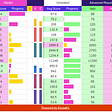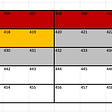# Infinite Data structures in Javascript

TL;DR. Looking at the dual infinite codata structures, we will create a structure like this :

`var stream = (1, (2, (3, (4, ...))));`

because we want to generate something infinite we will use (co)recursion to construct it like this :

`var f= n => (n, f(n + 1));console.log(f(0)) //"too much recursion"                                                                                                                                              run this: .NetFiddle                                         wikipedia:See corecursion`

so we use laziness

`var stream = (a,substream)=>({current:a ,next:substream}); var f = n => stream(n, ()=>f(n + 1)); console.log(fLazy(0).next().next().current) //2                                                run this: .NetFiddle`

# Dual of Data Structures. The Codata

Data structures like Lists, are the favorite data structure of programmers in any programming language. There are also the Opposite/Dual structures, that are not that well known. Usually they are infinite, and not that intuitive. Structures like Observables in Reactive extensions that have gained some traction finally. Lets see some basic ideas around co-data structures.

we will start from our default data structures.We all love the list. List’s Recursive definition is pretty linear :

`A list is either an element or a conacatenation of an element and a list`

in the above sentence if we replace the word element with a, the either with +, and the concat with * we get the algebraic definition :

`List a = a + a *(List a)`

if you see closer you will see the plus sign +. This is the logical or also known as Either. The + signifies the fact, that we can always terminate a list by taking [either] a plain` a` as our last element.

if we remove this option we can get something like this :

`Stream a =  a * (Stream a)`

[this * is the dual of the + above. Its a product structure that signifies something equivalent to logical And]

A structure can only be an `a` and a new Stream of a’s. There is no end in this structure. Its like [actually its the same] a recursive function that does not have a terminating condition. Stack-overflow.

Lets see an example of something called Co-free comonad that displays this property. [the Stream data structure above is actually isomorphic to the Cofree of the Identity functor thats why i mentioned it]

It looks like the stream above :

`Cofree(F,a) = (a, F(Cofree(F,a)))`

an example instance of a cofree-comonad would be this

`var comonad = coFree(2, Id(coFree(2, ...)));`

What this is saying is: “a cofree is a product of an `a `and a container (functor) of a cofree” for example if we take as F the identity “object” `Id=a=>({a:a})`(the simplest container) and as carrier type the integers, we could write an example instance of the associated cofree comonad:

`var comonad = coFree(1, Id(coFree(2, Id( coFree(3,… ))));`

the thing is that we have to always provide as a second argument something. So this actually does not terminate. Ever. So we possibly cannot even construct it. Well we can use recursion.

Ok we constructed it with ana but if we try to materialize it with ana(0) we get a nice “too much recursion” .

# Laziness

now we will use the lazy operation ()=>_ to delay the execution of the whole recursion.

`var ana= n => CoFreeF(n + 1, ()=> Identity( ana(n + 1)));`

it’s the same, but it does execute lazily. Only when we ask for a value.

we could also feed the Cofree with the lazy Identity Functor:

`var IdentityLazy =v =>({ v: ()=>v, });`

we can get the numbers in the sequence if we use next():

`var three = stream.next().value.next().value.a; //3var four = stream.next().value.next().value.next().value.a; //4`

If you reached here congrats you now understood the concept of generators in (ES6). Well kind of. I wanted to provide here a hint about the underlying maths and concepts. here a recursive cat for you.

# Stream as Functor

the structure of Cofree is a Simple recursive Tree and we know how to create a valid map on this algebraic data stracture

But there is more. I left the`Id=a=>({a:a})`in the example above in order to replace it with the identity functor (You can see more about functors here) this is a cofree comonad that has a map function that makes it a Functor. This map will take a function and apply it to all the elements of the stream. This is the implementation:

`var Id = v => ({v: v,map: f => Id(f(v)) });var CoFreeF = (a, next) => ({   a: a,   next: next,   extract: () => a,   map: g => CoFreeF(g(a), () => next().map(x=>x.map(g))), });var f = n => CoFreeF(n + 1, () => Id(f(n + 1)));`

In order to implement map and since it’s a recursive product type, we will return the first element mapped and then we will pass the function to the Id(_) functor inside, which is the second argument and let it recursively map its children [next().map(g)].

now we can write stuff like :

`var x = stream.next().next().map(x => x * 2).next();`

Exercise: Can you fork the JsFiddle and implement a Filter function that will return a filtered stream?

i will just say that this has something to do with the Observable mapping . Also the concept of co-routines is strongly related to those ideas. And also the concept of call-with-current-continuation [is isomorphic to the generator]

You can find more in my upcoming book Functional Programming in Javascript with Categories in LeanPub.

--

--

--

More than 14 years’ experience as full stack developer and Software Architect .https://leanpub.com/u/dimitrispapadim

Love podcasts or audiobooks? Learn on the go with our new app.

## What is REST API: a beginner’s guide## Web Development: The Static Fundamentals## Best Screen Recorder and Streaming app for Ubuntu; Download and Install OBS Studio on Ubuntu## What’s the difference between a “coder”, programmer, software developer, and software engineer?## Don’t Let Anyone Steal Your Domain Name Idea !## Aim Journey ⛰️- Week 10## Starting a New Group Project on GitHubMore than 14 years’ experience as full stack developer and Software Architect .https://leanpub.com/u/dimitrispapadim

## Data structures: Array## OOP 101 (Object-Oriented Programming)